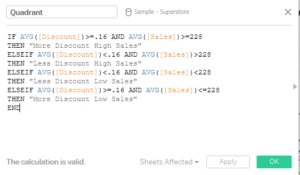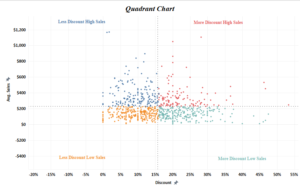Hi All,
In this blog of Advanced charting series we will take a look into how to build a quadrant chart in tableau . A Quadrant chart divides the chart area into four quadrants  : High-High , High-Low , Low-High and Low-Low.
To create a quadrant chart in tableau we will have to divide the chart area into 4 regions based on the measures value we have selected on X and Y axes.
Let move forward and create a Quadrant chart in tableau.

The basic requirement for a Quadrant Chart is a scatter plot . A scatter plot is a chart type in which two continuous variables are represented on two different axes.
To create a Scatter plot lets bring Discount in columns and Sales in Rows (used sample superstore data) . Select the default aggregation to average.
Bring Customer name to Detail.
Now lets go ahead and divide this scatter plot into 4 quadrants . To consider the values for the Quadrants we will consider the average of Sales and average of Discount.

Create a calculated field as under :Here I have taken as the Average discount value to be 16% and average sales per customer to be \$228.
Format the chart area to bring up the labels and markHope you guys followed it. Do let me know if this is not clear .

Thanks.
Rahul Singh